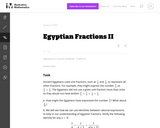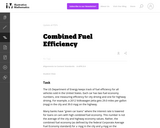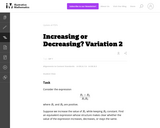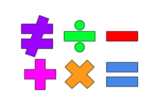Updating search results...

# 4 Results

View
Selected filters:
• UT.MATH.III.A.APR.6Unrestricted Use
CC BY
Rating
0.0 stars

This is a task from the Illustrative Mathematics website that is one part of a complete illustration of the standard to which it is aligned. Each task has at least one solution and some commentary that addresses important asects of the task and its potential use. Here are the first few lines of the commentary for this task: Ancient Egyptians used unit fractions, such as $\frac{1}{2}$ and $\frac{1}{3}$, to represent all other fractions. For example, they might express the n...

Subject:
Mathematics
Secondary Mathematics
Material Type:
Activity/Lab
Provider:
Illustrative Mathematics
Provider Set:
Illustrative Mathematics
Author:
Illustrative Mathematics
05/24/2013Unrestricted Use
CC BY
Rating
0.0 stars

The primary purpose of this problem is to rewrite simple rational expressions in different forms to exhibit different aspects of the expression, in the context of a relevant real-world context (the fuel efficiency of of a car). Indeed, the given form of the combined fuel economy computation is useful for direct calculation, but if asked for an approximation, is not particularly helpful.

Subject:
Mathematics
Secondary Mathematics
Material Type:
Activity/Lab
Provider:
Illustrative Mathematics
Provider Set:
Illustrative Mathematics
Author:
Illustrative Mathematics
01/25/2013Unrestricted Use
CC BY
Rating
0.0 stars

The purpose of this task is to help students see manipulation of expressions as an activity undertaken for a purpose.

Subject:
Mathematics
Secondary Mathematics
Material Type:
Activity/Lab
Provider:
Illustrative Mathematics
Provider Set:
Illustrative Mathematics
Author:
Illustrative Mathematics
05/01/2012Unrestricted Use
Public Domain
Rating
0.0 stars

This lesson is for a math classroom, but can be adapted to fit any grade, subject, or content.&nbsp;&nbsp;In this lesson, students will use an iPad and its features: Keynote, Pages, Garageband, Numbers, Presentation, and iMovie.&nbsp; Students will use 3 of those features/programs to create a video lesson consisting of several examples from a topic of their choice.&nbsp; This project is in place of a term final, so their chosen topic should be from their current term.Image citation: The image is one I created.&nbsp;&nbsp;

Subject:
Secondary Mathematics
Material Type:
Assessment
Author:
Tyrani Bevell